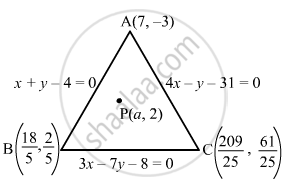Advertisement Remove all ads

# Find the Values of the Parameter a So that the Point (A, 2) is an Interior Point of the Triangle Formed by the Lines X + Y − 4 = 0, 3x − 7y − 8 = 0 and 4x − Y − 31 = 0. - Mathematics

Answer in Brief

Find the values of the parameter a so that the point (a, 2) is an interior point of the triangle formed by the lines x + y − 4 = 0, 3x − 7y − 8 = 0 and 4x − y − 31 = 0.

Advertisement Remove all ads

#### Solution

Let ABC be the triangle of sides AB, BC and CA whose equations are  x + y − 4 = 0, 3x − 7y − 8 = 0 and 4x − y − 31 = 0, respectively.
On solving them, we get

$A \left( 7, - 3 \right)$,

$B \left( \frac{18}{5}, \frac{2}{5} \right)$  and $C \left( \frac{209}{25}, \frac{61}{25} \right)$  as the coordinates of the vertices.
Let P (a, 2) be the given point.It is given that point P (a,2) lies inside the triangle. So, we have the following:
(i) A and P must lie on the same side of BC.
(ii) B and P must lie on the same side of AC.
(iii) C and P must lie on the same side of AB.
Thus, if A and P lie on the same side of BC, then $\left( 21 + 21 - 8 \right)\left( 3a - 14 - 8 \right) > 0$

$\Rightarrow a > \frac{22}{3}$         ... (1)

If B and P lie on the same side of AC, then $\left( \frac{4 \times 18}{5} - \frac{2}{5} - 31 \right)\left( 4a - 2 - 31 \right) > 0$

$\Rightarrow a < \frac{33}{4}$       ... (2)

If C and P lie on the same side of AB, then

$\left( \frac{209}{25} + \frac{61}{25} - 4 \right)\left( a + 2 - 4 \right) > 0$

$\Rightarrow \left( \frac{34}{5} - 4 \right)\left( a + 2 - 4 \right) > 0$

$\Rightarrow a > 2$       ... (3)
From (1), (2) and (3), we get:

$a \in \left( \frac{22}{3}, \frac{33}{4} \right)$

Is there an error in this question or solution?
Advertisement Remove all ads

#### APPEARS IN

RD Sharma Class 11 Mathematics Textbook
Chapter 23 The straight lines
Exercise 23.14 | Q 2 | Page 102
Advertisement Remove all ads

#### Video TutorialsVIEW ALL 

Advertisement Remove all ads
Share
Notifications

View all notifications

Forgot password?